# Persistent State and Cursor in Oracle

## Persistent State and Cursor in Oracle

In this article, I am going to discuss Persistent State and Cursor in Oracle with Examples. Please read our previous article where we discussed PRAGMA SERIALLY_REUSABLE in Oracle. In this article, we will try to learn the advantages of the persistent state and how it works inside the packages.

```create or replace package cur_pkg
is
cursor c_emp is
select employee_id from employees;

procedure open;
procedure close;
procedure printnext_20;
end;
```

We have created the package specification with the name cur_pkg. The package specification contains a cursor that takes the selected employee_id from the table employees. This cursor will retrieve the employee_id from the employees table.

We have three procedures where the first procedure opens the cursor and second procedure will close the procedure and the third procedure will print the next 20 rows in the table. So, each time when we execute this procedure it will print the next 20 employees. Let’s compile the package specification.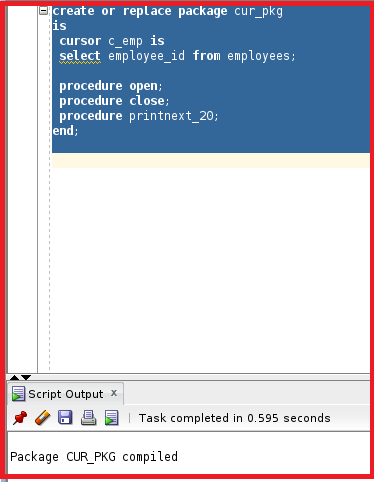So, the package specification is now compiled. Let’s have a look at the package body.

```create or replace package body cur_pkg is
procedure open
is
begin
if not c_emp%isopen then
open c_emp;
end if;
end open;
procedure close
is
begin
if c_emp%isopen then
close c_emp;
end if;
end close;
procedure printnext_20
is
v_emp_id number;
begin
for i in 1..20
loop
fetch c_emp into v_emp_id;
dbms_output.put_line(v_emp_id);
if c_emp%notfound then dbms_output.put_line('no more');
close c_emp;
exit;
end if;
end loop;
end printnext_20;
end cur_pkg;
```

This is the package body. This package body has the code of procedure open, procedure close, and procedure print_next20. Let’s try to look at each procedure and see how this works.

###### Procedure Open:
```procedure open
is
begin
if not c_emp%isopen then
open c_emp;
end if;
end open;
```

The procedure open contains the code as if the variable c_emp is not open then open the cursor variable. So, practically, if the cursor is not open, we can open the cursor. In the end, we have mentioned the end open. We can use the procedure name at the end.

###### Procedure Close:
```procedure close
is
begin
if c_emp%isopen then
close c_emp;
end if;
end close;
```

This procedure show if the procedure is open then close the procedure. The variable cursor c_emp is open then close the cursor. Let’s have a look at the procedure printnext_20.

```procedure printnext_20
is
v_emp_id number;
begin
for i in 1..20
loop
fetch c_emp into v_emp_id;
dbms_output.put_line(v_emp_id);
if c_emp%notfound then dbms_output.put_line('no more');
close c_emp;
exit;
end if;
end loop;
end printnext_20;
```

This procedure contains v_emp_id as a new variable. We have mentioned the for loop from 1 to 20 for variable ‘I’. For the cursor we need a loop. The value of v_emp_id will be fetched from c_emp when the cursor is open. The value of v_emp_id will be used in dbms_output. If c_emp is not found then print “no more”. So, let’s try to execute the package body.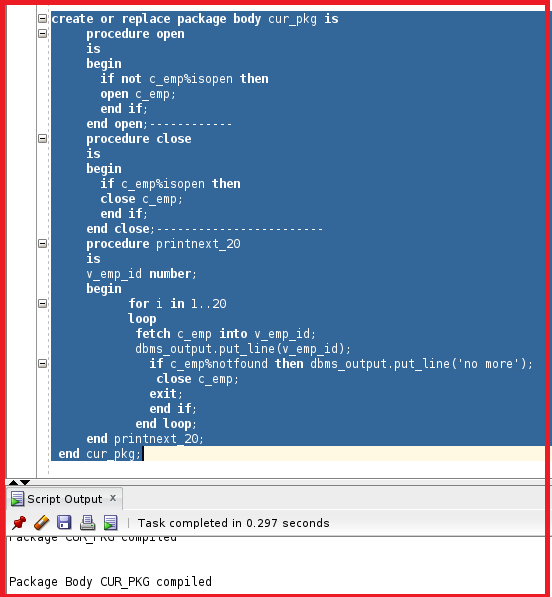So, the package body is now compiled. Let’s try to test the package and the cursor.

```begin
cur_pkg.open;
cur_pkg.printnext_20;
end;
```

So, in this statement, we are opening the cursor and printing the next 20 variables. Let’s execute this statement.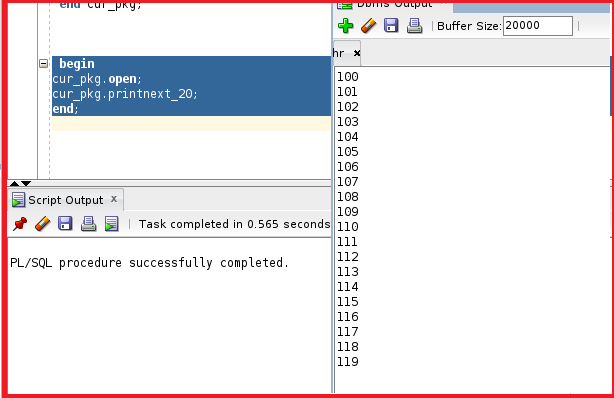We can see the statement is executed and the DBMS output prints the first 20 employee_ids. The procedure will first open and then the procedure printnext_20 will print the next 20 employee_id. The table is not finished yet, there are still more rows present inside the table. The cursor is still open inside the memory, so the persistent values will be present and when we try to execute again it takes the current values and prints the next 20 values. So, let’s try to execute again.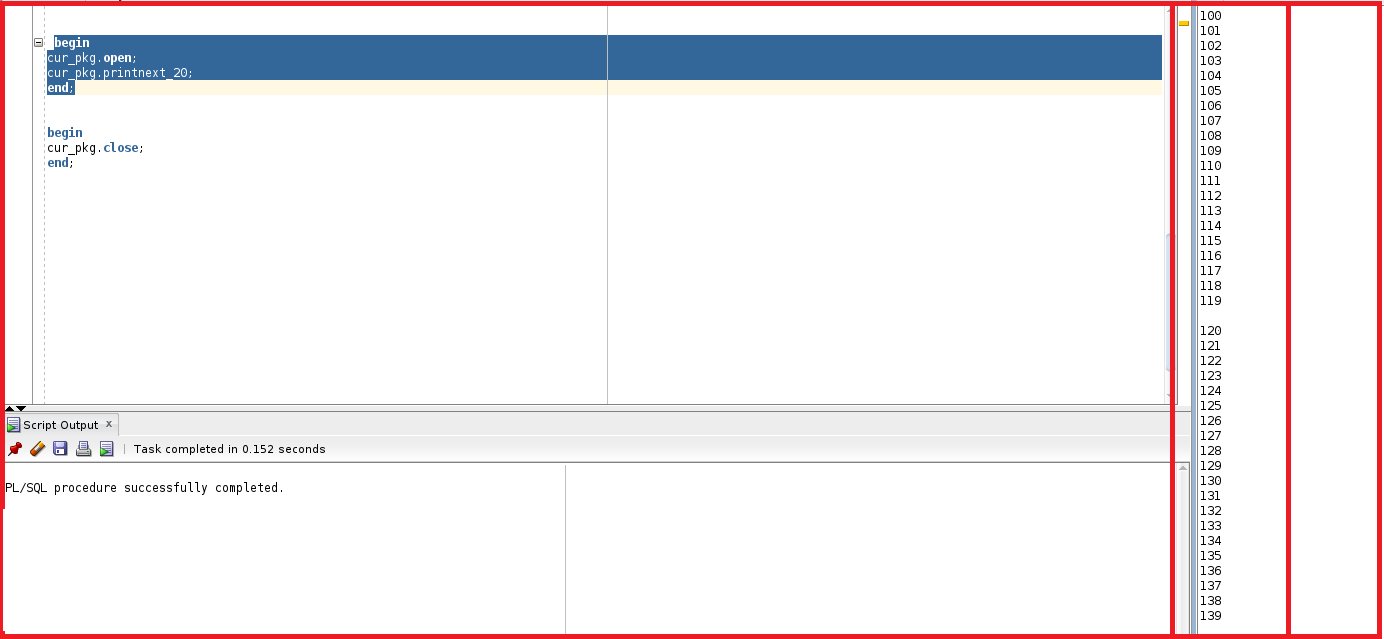We can see the values are moved from 120 to 139. So, the current value is  at 139. Let’s try to execute the package again till the end.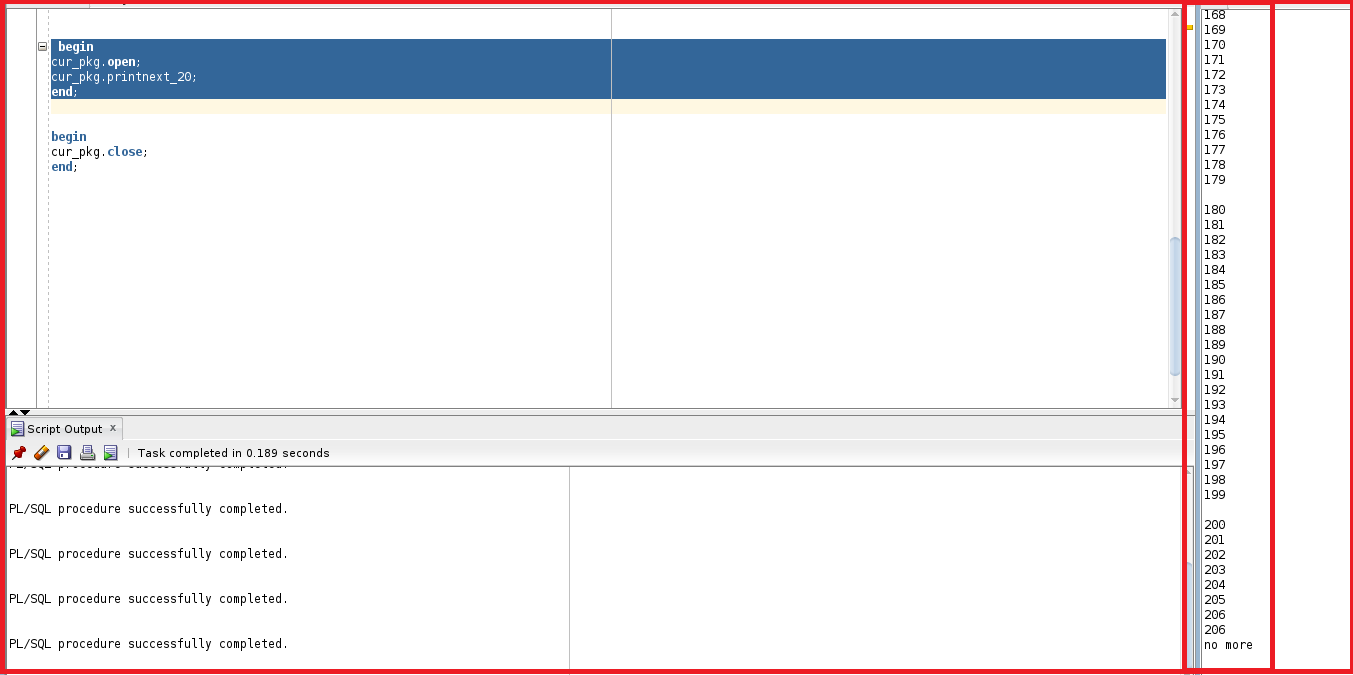In the end, we can see no more. So, after executing the package multiple times. We found “no more”. So, here we are at the end of the table and the cursor will be closed automatically. So, we understood the benefits of the persistent state and the cursor as well using the procedure. Let’s try to close the procedure.

```Begin
cur_pkg.close;
end;
```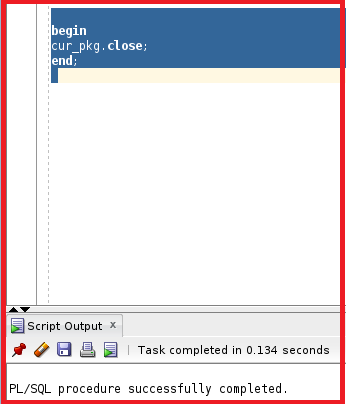Note: This code will work only with packages. If we try to execute this concept with only procedure it will never work because the persistent works only with packages.

In the next article, I am going to discuss Oracle Supplied Packages with Examples. Here, in this article, I try to explain Persistent State and Cursor in Oracle with Examples. I hope you enjoy this Persistent State and Cursor in Oracle article.